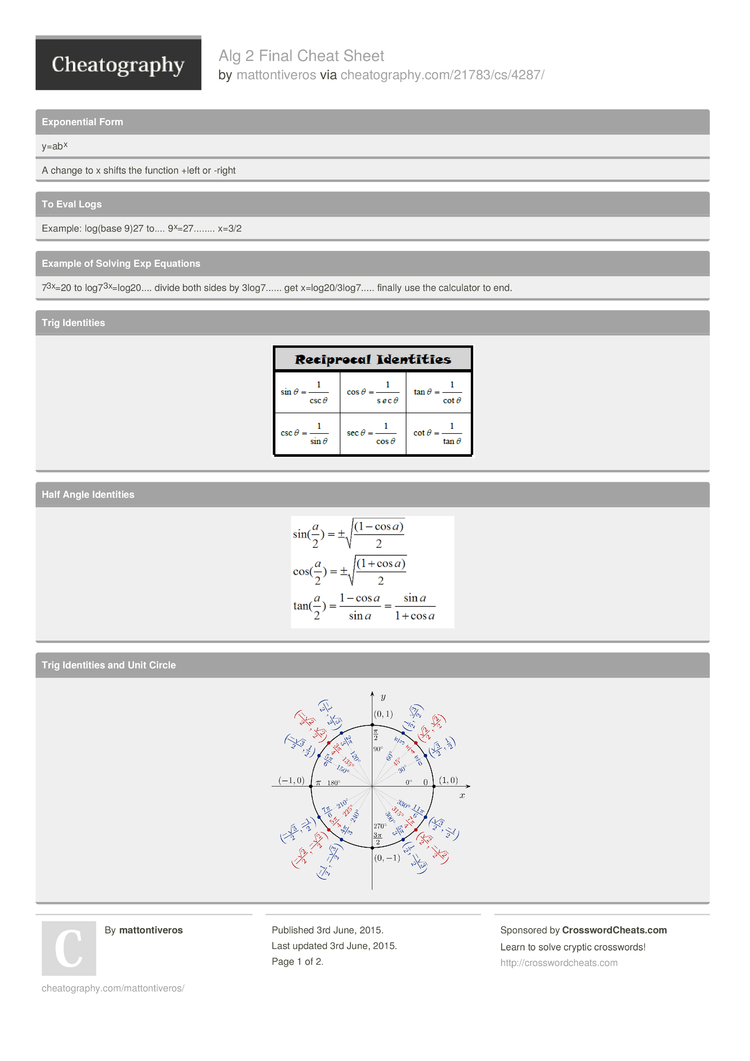# Alg 2 Final Cheat Sheet by mattontiveros

### Expone­ntial Form

 y=abx
A change to x shifts the function +left or -right

### To Eval Logs

 Example: log(base 9)27 to.... 9x=2­7........ x=3/2

### Example of Solving Exp Equations

 73x=20 to log73­x=­log­20.... divide both sides by 3log7...... get x=log2­0/3­log­7..... finally use the calculator to end.

### Trig Identities### Half Angle Identities### Trig Identities and Unit Circle### Double Angle### Note for Linear Modeling

 Find line of best fit, f(x) is the prediction of that number plugged into formula.

### Properties of Parabolas Note

 Find AOS first; x=number of AOS

### Identities

 cscx= 1/sinx secx=1­­/cosx cotx=1­­/tanx tanx=s­­in­x­/cosx cotx=c­­os­x­/sinx2 Pages
//media.cheatography.com/storage/thumb/mattontiveros_alg-2-final.750.jpg

PDF (recommended)